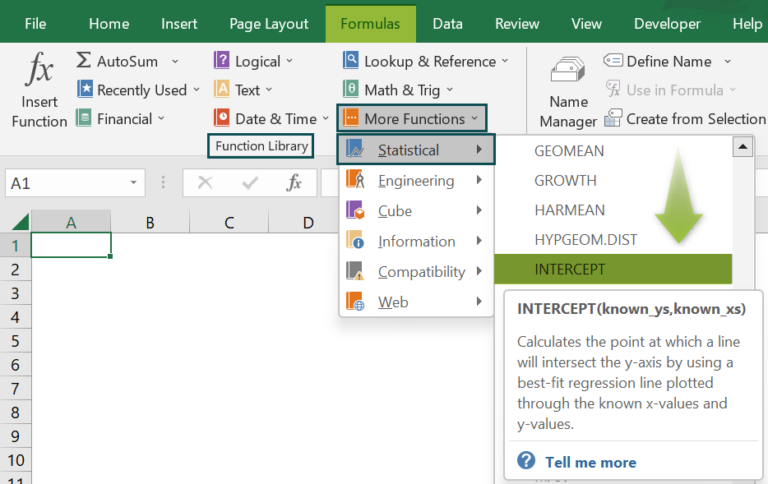# INTERCEPT Excel FunctionArticle byExcelMojo Team## What Is INTERCEPT Function In Excel?

The INTERCEPT Excel function evaluates the point at which a line will intersect the y-axis using existing x-values and y-values. The INTERCEPT function is an inbuilt Statistical function, so we can insert the formula from the “Function Library” or enter it directly in the worksheet.

For example, we will apply the INTERCEPT function to calculate the output of two sets of values in columns A and B.

Select cell B5, enter the formula =INTERCEPT(A2:A4,B2:B4), and press “Enter”. The result is ‘3.071’, also, the graphical representation is shown below.

###### Key Takeaways
• The INTERCEPT Excel function calculates the intersecting point of a line using the values of x and y, when the selected array is same in size.
• In a selected cell range, if one of the x-value and its corresponding y-value is blank, we will get an INTERCEPT result, however, we cannot plot a graph.
• An argument containing text, logical values, or empty cells are ignored while calculating the INTERCEPT.
• If one of the cell values is 0, it is still included in the calculation.

### INTERCEPT() Excel Formula

The syntax of the INTERCEPT Excel formula is,

The arguments of the INTERCEPT Excel formula are,

• known_ys: It is a mandatory argument. These are the “known_ys” values to calculate the intersection.
• known_xs: It is a mandatory argument. These are the “known_xs” values to calculate the intersection.

### How To Use INTERCEPT Excel Function?

We can use the INTERCEPT Excel function in 2 ways, namely,

1. Access from the Excel ribbon.
2. Enter in the worksheet manually.

#### Method #1 – Access from the Excel ribbon

Choose an empty cell for the output → select the “Formulas” tab → go to the “Function Library” group → click the “More Function” option drop-down → click the “Statistical” option right arrow → select “INTERCEPT” function, as shown below.

The “Function Arguments” window appears. Enter the arguments in the Known_ys”, and the Known_xsfields → click “OK”, as shown below.

#### Method #2 – Enter in the worksheet manually

1. Select an empty cell for the output.
2. Type =INTERCEPT( in the selected cell. [Alternatively, type =I or =IN and double-click the INTERCEPT function from the list of suggestions shown by Excel.]
3. Enter the arguments as cell values or Excel cell references and close the brackets.
4. Press the “Enter” key.

Lets’s take a basic example to learn its application.

We will calculate the output using INTERCEPT Excel function for the value of x and y.

In the table, the data is,

• Column A contains the ‘x’ values.
• Column B contains the ‘y’ values.

The steps to calculate the regression using INTERCEPT formula are,

1. Select cell B7, and enter the formula =INTERCEPT(B2:B6, i.e., the value of the known_ys.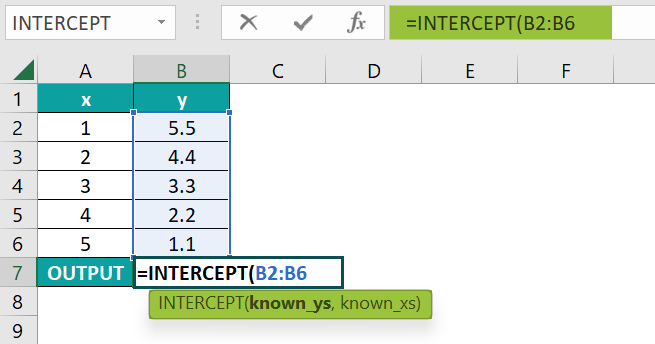2. Enter the value of the known_xs argument as “A2:A6”, and close the brackets. The complete formula is =INTERCEPT(B2:B6,A2:A6)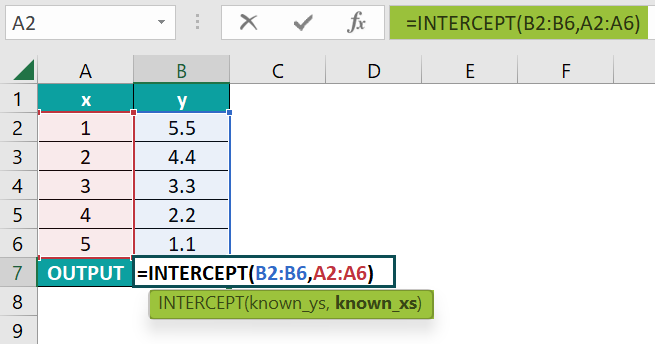3. Press the “Enter” key. The result is “6.6”, and the graphical representation is shown below.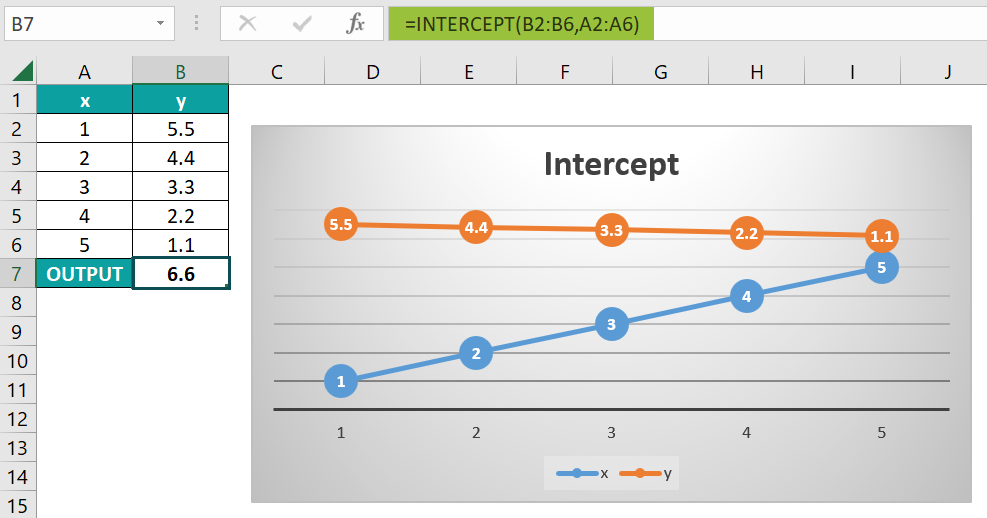### Examples

We will understand some advanced scenarios using the INTERCEPT Excel function examples.

#### Example #1

We will calculate the output using the INTERCEPT function with a line graph for the value of x and y.

In the table, the data is,

• Column A contains the ‘x’ values.
• Column B contains the ‘y’ values.

The procedure to calculate the regression by the INTERCEPT formula is,

Select cell B7, enter the formula =INTERCEPT(B2:B6,A2:A6), and press the “Enter” key. The calculated result is “-0.08”, as shown in the following image with a line graph.

#### Example #2

We will calculate the output using the INTERCEPT function for the monthly sales & quantity.

In the table, the data is,

• Column A contains the Months.
• Column B contains the Sales.
• Column C contains the Quantity.

The procedure to calculate the regression by the INTERCEPT formula is,

Select cell C14, enter the formula =INTERCEPT(B2:B13,C2:C13), and press the “Enter” key. The calculated result is “-47.582”, as shown below, also the graphical representation.

#### Example #3

We will calculate the output using the INTERCEPT function for the time & distance values.

In the table, the data is,

• Column A contains the Time in Seconds.
• Column B contains the Distance in Meters.

The procedure to calculate the regression by the INTERCEPT formula is,

Select cell C14, enter the formula =INTERCEPT(A2:A5,B2:B5), and press the “Enter” key. The calculated result is “4.7052”, as shown below, also the graphical representation.

### Important Things To Note

• The “#N/A!” error occurs when the argument’s cell range is not of the same size.
• The arguments consider numbers or names, arrays, or cell references that contain numeric values.
• The “#DIV/0!” error occurs when there is only one x and y-value in a given dataset.

1. What does the INTERCEPT Excel function mean?

The INTERCEPT Excel function is a regression line plotted as a result of the intersection based on known x and y-values. It calculates the value of the dependent variable when the independent variable is equal to zero.

Syntax of the INTERCEPT function is =INTERCEPT(known_ys,known_xs)
We will calculate the output using the INTERCEPT() for the time & distance values.

In the table, the data is,
Column A contains the known_ys.
Column B contains the known_xs.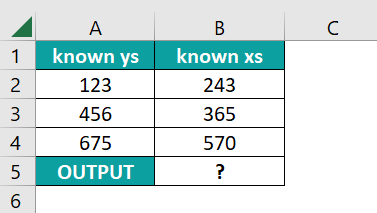The procedure to calculate the regression by the INTERCEPT formula is,

Select cell C14, enter the formula =INTERCEPT(A2:A4,B2:B4), and press the “Enter” key. The calculated result is “-219.576”, as shown below, also the graphical representation.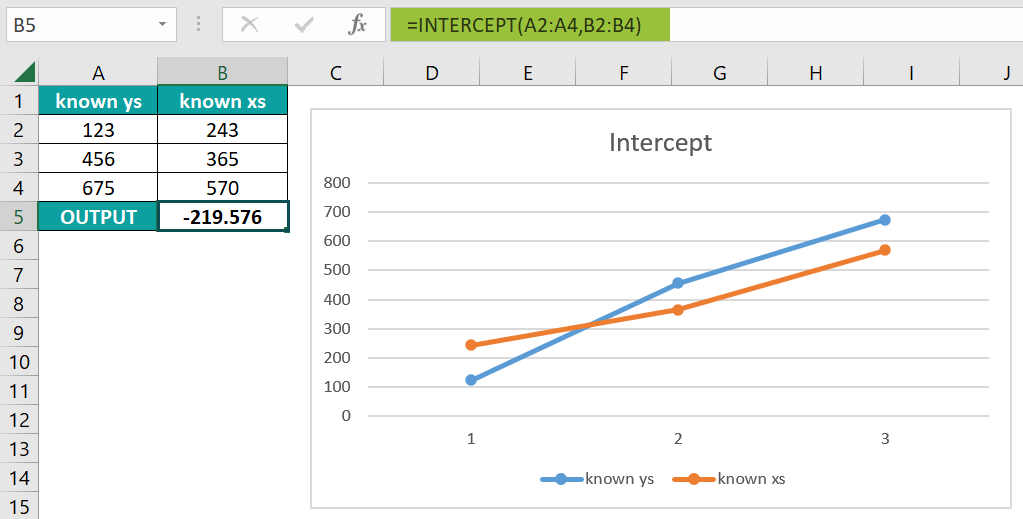2. How to insert the INTERCEPT Excel function?

We can insert the INTERCEPT function as follows:

1. Select an empty cell for the output.
2. Type =INTERCEPT( in the selected cell. [Alternatively, type =I or =IN and double-click the INTERCEPT function from the list of suggestions shown by Excel.]
3. Enter the arguments as cell values or cell references and close the brackets.
4. Press the “Enter” key.

For example, the image depicts the time & distance values in columns A & B. We will calculate the output using the INTERCEPT function.
In the table, the data is,

Column A contains the known_ys.
Column B contains the known_xs.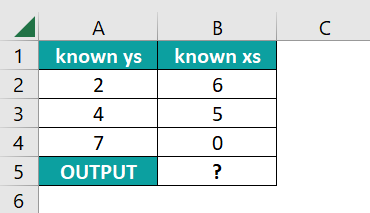The procedure to calculate the regression by the INTERCEPT formula is,

Select cell C14, enter the formula =INTERCEPT(A2:A4,B2:B4), and press the “Enter” key. The calculated result is “7.1129”, as shown below, also the graphical representation.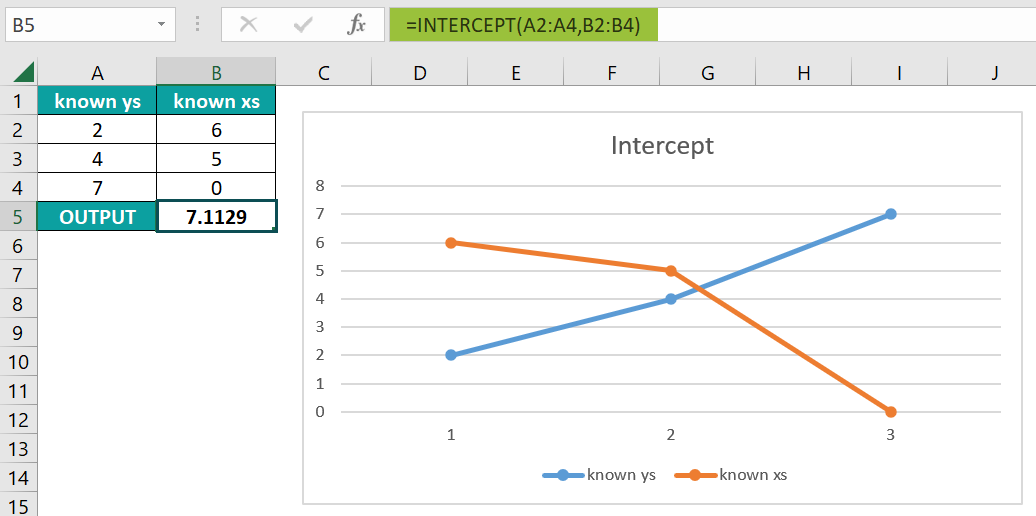3. Where is the INTERCEPT Excel function?

The INTERCEPT function is found in the “Formulas” tab, as shown below.

Choose an empty cell for the output → select the “Formulas” tab → go to the “Function Library” group → click the “More Function” option drop-down → click the “Statistical” option right arrow → select “INTERCEPT” function, as shown below.